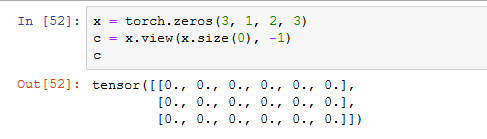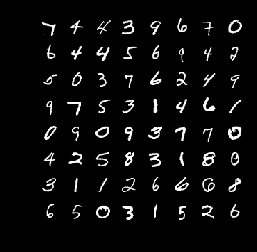# 关于pytorch的一些笔记

Posted by kevin on March 20, 2020

## unsqueeze

tensor.unsqueeze(tensor, dim=x) 用来给 tensor 升维，也就是加上一个维数为 1 的维度（dim 参数不能超过 tensor 的维度），例如

import torch
a = torch.rand((1, 2, 3))
a.size()
# torch.Size([1, 2, 3])
b = torch.unsqueeze(a, 2)
b.size()
# torch.Size([1, 2, 1, 3])


unsqueeze 这个函数还是挺常用的，例如在处理逻辑回归时输入的点为一维的数据，我们就要用 unsqueeze 来升维使其变成二维的数据。同理，tensor.squeeze(tensor, dim=x) 就是用来降维的，如果不指定 dim 参数的话就默认将所有维数为 1 的维度都删除

import torch
a = torch.rand((1, 1, 2, 3))
a.size()
# torch.Size([1, 1, 2, 3])
b = torch.squeeze(a)
b.size()
# torch.Size([2, 3])


import torch
a = torch.rand((1, 1, 2, 3))
a.size()
# torch.Size([1, 1, 2, 3])
b = torch.squeeze(a, 2)
b.size()
# torch.Size([1, 1, 2, 3])
c = torch.squeeze(a, 1)
c.size()
# torch.Size([1, 2, 3])## visualization

import cv2
import matplotlib.pyplot as plt
plt.imshow(cv2.cvtColor(img, cv2.COLOR_BGR2RGB))
plt.show()


def imshow(img):
npimg = img.numpy()
plt.axis('off')
plt.imshow(np.transpose(npimg, (1, 2, 0)))

images, labels = dataiter.next()torchvision.utils.save_image(tensor, fp, format, normalize=True) 可以将一个 batch 的图片给保存下来，因为这里面直接会调用 make_grid 函数，跟上面是一样的效果

• tensor (Tensor or list) – Image to be saved. If given a mini-batch tensor, saves the tensor as a grid of images by calling make_grid.
• fp (string or file object) – A filename or a file object
• format (Optional) – If omitted, the format to use is determined from the filename extension. If a file object was used instead of a filename, this parameter should always be used.
• **kwargs – Other arguments are documented in make_grid.
import time
import torchvision.utils as vutils
vutils.save_image(data['img'].data, 'images/{}.png'.format(time.time()), nrow=4, padding=0, normalize=True)


import time
import matplotlib.pyplot as plt
item = torch.mean(x, dim=1).detach().cpu().numpy().transpose((1,2,0))
plt.figure()
plt.axis('off')
plt.imshow(item,cmap='jet')
plt.savefig(f'fig{time.time()}.png')


## optimizer

optimizer = optim.SGD([{'params':models.parameters() ,'lr': 0.0001},
{'params':models.parameters()},
{'params':models.parameters()}],
lr=opt.learning_rate,
momentum=opt.momentum,
weight_decay=opt.weight_decay)


optimizer = optim.SGD([model.parameters() for model in models],
lr=opt.learning_rate,
momentum=opt.momentum,
weight_decay=opt.weight_decay)


## GPU

device = torch.device('cuda' if torch.cuda.is_available() else 'cpu')
data.to(device)


import os
#多块使用逗号隔开
os.environ['CUDA_VISIBLE_DEVICES'] = '6'


$CUDA_VISIBLE_DEVICES=6 python xx_train.py  ## loss_function loss_function 有些在 torch.nn.functionaltorch.nn 里面都有，但是调用起来的方法是不一样的，而且一个需要大写首字母，一个不需要。具体内容看以下代码 import torch.nn as nn criterion = nn.CrossEntropyLoss() loss = criterion(out, label) loss = nn.CrossEntropyLoss()(out, label) # 或者直接这样写  import torch.nn.functional as F loss = F.cross_entropy(out, label)  ## train&test 测试的时候要将 model 变成 eval 模式（net.eval()），一般是在 train 模式的，如果测试后还要接着训练的话在最后加上 net.train() 继承了 nn.Module 的类都有一个属性叫做 self.training ，通过判断这个性质就可以知道网络是否处于训练状态，一般用 net.train()net.eval() 就是对这个属性进行修改 测试时用 with xx.no_grad() 不计算梯度，减小显存开销和算力。如果不在意显存开销的话用 model.eval() 就够了，这就可以改变 Batch Normalization 和 Dropout 的行为。eval() 模式的梯度计算与储存和 train() 一样，只不过不会反向传播更新参数罢了。 ## save&load 加载 load 训练好的模型的时候要将模型的定义代码一起包含进来，否则会报错说找不到网络结构 当不需要对变量进行梯度更新时，可以在后面加上 .detach() ，这样做相当于将 requre_grad 置为 False，在 GAN 中固定 Discriminator 时会用到这个 加载预训练好的模型使用 torch.load(model_path) ，这样得到的是一个字典，保存了网络的每一个参数的 key 和 value，但是有时候我们可能会对网络进行更改，然后模型的参数就对不上了，可能相比原来会多一些参数或少一些参数，然后我们就要在 load 的时候选择 strict=False， 否则的话就会报错。用了 strict=False 的话，如果网络参数和模型参数有相同的 key 就会给该 key 赋值，没有的话就不会去管它 state_dict = torch.load('last.pth') base_model.load_state_dict(state_dict, strict=False)  如果模型在训练的时候使用了 nn.DataParallel 的话，保存的模型文件前面的 key 都会有个 module. 前缀，导入进来的话要么也对网络使用 nn.DataParallel ，要么就将模型参数改一下，将 module. 前缀去掉 state_dict = torch.load('last.pth') model.load_state_dict({k.replace('module.', ''): v for k, v instate_dict.items()})  ## torch.max torch.max(tensor, dim=x) 返回的是 tensor 中的最大值以及最大值的索引号，dim 参数表示取的是横向的还是竖向的最大值，0 代表每个纵向的最大值，1 代表每个横向的最大值 import torch torch.manual_seed(1) a = torch.rand(3, 4) value, index = torch.max(a, dim=1) print('{}\n{}\n{}'.format(a, value, index)) # tensor([[0.7576, 0.2793, 0.4031, 0.7347], # [0.0293, 0.7999, 0.3971, 0.7544], # [0.5695, 0.4388, 0.6387, 0.5247]]) # tensor([0.7576, 0.7999, 0.6387]) # tensor([0, 1, 2])  经常在神经网络的分类任务求准确率的时候用到这个函数，要记住的是 max 函数有两个返回值，并且也要知道 dim 代表的含义，在 torch.nn.functional.softmax(tensor, dim=x) 中的 dim 跟这里的 dim 也是有一样的含义 ## torch.manual_seed(x) torch.manual_seed(x) 用来固定随机数，使得每次生成的随机数都是相同的，常用来复现他人结果 $v = \frac{v}{\max(\lVert v \rVert_p, \epsilon)}$ ## torch.cat & torch.stack torch.cat 和 torch.stack 都可以将两个 tensor 连接成一个，但是用法有点不同，以 pytorch 经常处理的四维 tensor 来举例子 ### torch.cat 简单的说， cat 会将两个 tensor 中指定维度的数据堆在一起，扩充该维度的大小，要求两个 tensor 的维度必须一致，并且经过 cat 后的 tensor 的维度不会变，和之前一样。下面看看例子 import torch a0 = torch.Tensor([[[[1,1,1,1],[2,2,2,2]]]]) a1 = torch.Tensor([[[[3,3,3,3],[4,4,4,4]]]]) l = [] l.append(torch.cat((a0,a1),dim=0)) l.append(torch.cat((a0,a1),dim=1)) l.append(torch.cat((a0,a1),dim=2)) l.append(torch.cat((a0,a1),dim=3)) for i in l: print('{}\n{}\n{}\n\n\n'.format(i, a1.size(), i.size()))  分别在四个维度上做实验，得到的结果如下： tensor([[[[1., 1., 1., 1.], [2., 2., 2., 2.]]], [[[3., 3., 3., 3.], [4., 4., 4., 4.]]]]) torch.Size([1, 1, 2, 4]) torch.Size([2, 1, 2, 4]) tensor([[[[1., 1., 1., 1.], [2., 2., 2., 2.]], [[3., 3., 3., 3.], [4., 4., 4., 4.]]]]) torch.Size([1, 1, 2, 4]) torch.Size([1, 2, 2, 4]) tensor([[[[1., 1., 1., 1.], [2., 2., 2., 2.], [3., 3., 3., 3.], [4., 4., 4., 4.]]]]) torch.Size([1, 1, 2, 4]) torch.Size([1, 1, 4, 4]) tensor([[[[1., 1., 1., 1., 3., 3., 3., 3.], [2., 2., 2., 2., 4., 4., 4., 4.]]]]) torch.Size([1, 1, 2, 4]) torch.Size([1, 1, 2, 8])  ### torch.stack stack 会在拼接之前先将 tensor 给扩大一维，然后再将指定维度上的数据进行连接，也就相当于在这个例子中 dim 可以指定为 4（不过没人会这么用），如下： import torch a0 = torch.Tensor([[[[1,1,1,1],[2,2,2,2]]]]) a1 = torch.Tensor([[[[3,3,3,3],[4,4,4,4]]]]) l = [] l.append(torch.stack((a0,a1),dim=0)) l.append(torch.stack((a0,a1),dim=1)) l.append(torch.stack((a0,a1),dim=2)) l.append(torch.stack((a0,a1),dim=3)) l.append(torch.stack((a0,a1),dim=4)) for i in l: print('{}\n{}\n{}\n\n\n'.format(i, a1.size(), i.size()))  实验的结果如下，细细品，dim 指定哪个维度就在哪个维度前面加一个维数为 2 的维度 tensor([[[[[1., 1., 1., 1.], [2., 2., 2., 2.]]]], [[[[3., 3., 3., 3.], [4., 4., 4., 4.]]]]]) torch.Size([1, 1, 2, 4]) torch.Size([2, 1, 1, 2, 4]) tensor([[[[[1., 1., 1., 1.], [2., 2., 2., 2.]]], [[[3., 3., 3., 3.], [4., 4., 4., 4.]]]]]) torch.Size([1, 1, 2, 4]) torch.Size([1, 2, 1, 2, 4]) tensor([[[[[1., 1., 1., 1.], [2., 2., 2., 2.]], [[3., 3., 3., 3.], [4., 4., 4., 4.]]]]]) torch.Size([1, 1, 2, 4]) torch.Size([1, 1, 2, 2, 4]) tensor([[[[[1., 1., 1., 1.], [3., 3., 3., 3.]], [[2., 2., 2., 2.], [4., 4., 4., 4.]]]]]) torch.Size([1, 1, 2, 4]) torch.Size([1, 1, 2, 2, 4]) tensor([[[[[1., 3.], [1., 3.], [1., 3.], [1., 3.]], [[2., 4.], [2., 4.], [2., 4.], [2., 4.]]]]]) torch.Size([1, 1, 2, 4]) torch.Size([1, 1, 2, 4, 2])  reference: https://zhuanlan.zhihu.com/p/70035580 ## format_transform 使用 PIL 的 Image.fromarray 创建图象时，要求 numpy 数组的格式为 uint8 类型 to_tensor 是 pytorch 的 transforms 中的方法，将 PIL 格式的图片转化成 tensor 格式，原理是：PIL 储存图片的格式为（HWC），而 PIL 储存的是 （HWC），通过变换通道后，再将像素的值除以 255 得到 tensor。反过来，tensor 变成 PIL 格式的话就使用 transforms.ToPILImage() 方法 PIL 图像在转化成 numpy.ndarray 后，格式为（HWC），通道顺序是 RGB，用 Image.size 方法返回的是（W，H）。OpenCV 读入图片的格式就是 ndarray，格式为（HWC），通道顺序是 BGR 用 PIL 的 Image.Open(path) 读 png 图片只有一个通道，OpenCV 读到的是 3 个通道，不过会报错。所以如果拿 png 格式的图片训练的模型，在测试阶段用 jpg 格式去测试的话很可能会报错，因为 channel 数不对，解决办法就是判断图片的通道数（用 len(Image.split()) 来判断），如果 len 大于 1 的话说明不止一个通道，可以只取一个通道（如绿色通道）来进行测试。 有时候需要改变 tensor 的 type，可以用 tensor.dtype 来查看 tensor 的 type，例如 torch.int64 就是一种 type，然后如果要转化的话，用 tensor.to(type) 来进行，例如 tensor.to(torch.float32)，而不是像 numpy 一样用 array.astype(type) ## model_structure ### torch.nn.AdaptiveAvgPool2d() 自适应的平均池化，即只需要给定最终想要获得的 feather map 的 size 就行了，不用管怎么实现，并且通道数前后不变 e.g. torch.nn.AdaptiveAvgPool2d((1, 1)) 无论给定的输入 feather map 是多少，最终都会变成 (1 x 1) 的 feather map ### torch.nn.MaxPool2d() 参数列表为 kernel_size, *stride=None, padding=0, dilation=1, return_indices=False, ceil_mode=False，一般前两个参数都是 (2,2)，这里说一下 ceil_mode 这个参数，默认情况下是 False，用的是 floor_mode，feather map 的 output 按照下面这个公式计算 $$output = floor((W-K+2P)/S) + 1$$ 也就是向下取整，如果用了 ceil_mode 的话就是向上取整，公式如下 $$output = ceil((W-K+2P)/S) + 1$$ 有什么不同呢，会影响最后生成的 feather map 的尺寸，通过一个例子看看 import torch.nn as nn import torch x = torch.tensor([ [-2, 1, 2, 6, 4], [-3, 1, 7, 2, -2], [-4, 2, 3, -1 , -3], [-7, 1, 2, 3, 11], [5, -7, 8, 12, -9] ]).float() x = x.unsqueeze(0) y_1 = nn.MaxPool2d(kernel_size=2,stride=2, padding=0) y_2 = nn.MaxPool2d(kernel_size=2,stride=2, padding=0, ceil_mode=True) print(y_1(x)) print(y_2(x))  最终结果如下，所以用了 ceil_mode 的话相当于 padding 了几个 0 进行池化操作 tensor([[[1., 7.], [2., 3.]]]) tensor([[[ 1., 7., 4.], [ 2., 3., 11.], [ 5., 12., -9.]]]) ## torch.meshgrid 在 Faster RCNN 中有用到这个 API 用来将 anchor 从 feature map 上偏移到原图上，其实这个函数就是用来生成两个矩阵，在 Faster RCNN 中表示 anchor 在 x 和 y 方向上的偏移量（注意，numpy 也有 meshgrid，但是两者的顺序有不一样，详见我的 numpy 参考手册） shifts_x = torch.arange(0, 4, dtype=torch.float32) * 16 shifts_y = torch.arange(0, 4, dtype=torch.float32) * 16 shift_y, shift_x = torch.meshgrid(shifts_y, shifts_x) shift_x tensor([[ 0., 16., 32., 48.], [ 0., 16., 32., 48.], [ 0., 16., 32., 48.], [ 0., 16., 32., 48.]]) shift_y tensor([[ 0., 0., 0., 0.], [16., 16., 16., 16.], [32., 32., 32., 32.], [48., 48., 48., 48.]])  ## torch.where torch.where(condition, x, y) → Tensor 其实就是个三目表达式，根据 condition 的真假来决定该处的值用 x 还是 y，为真则用 x ，为假则用 y，将 x 和 y 通过这种方式结合在一起 >>> x = torch.randn(3, 2) >>> y = torch.ones(3, 2) >>> x tensor([[-0.4620, 0.3139], [ 0.3898, -0.7197], [ 0.0478, -0.1657]]) >>> torch.where(x > 0, x, y) tensor([[ 1.0000, 0.3139], [ 0.3898, 1.0000], [ 0.0478, 1.0000]]) >>> x = torch.randn(2, 2, dtype=torch.double) >>> x tensor([[ 1.0779, 0.0383], [-0.8785, -1.1089]], dtype=torch.float64) >>> torch.where(x > 0, x, 0.) tensor([[1.0779, 0.0383], [0.0000, 0.0000]], dtype=torch.float64)  如果 where 里面只有一个参数的话，返回的就是使 condition 为真的元素的索引 >>> input = torch.tensor([[0,1,2],[3,4,5],[6,7,8]]) >>> torch.where(input) (tensor([0, 0, 1, 1, 1, 2, 2, 2]), tensor([1, 2, 0, 1, 2, 0, 1, 2]))  这个例子就是返回 input 大于 0 的索引，有 8 个，分别是 (0,1) (0,2) (1,0) …… ## torch.unbind torch.unbind(input, dim=0) -> seq 用来移除某一个维度，返回的是一个 tuple ，元素个数为被移除的维度大小 >>> a = torch.tensor([[1,2,3],[4,5,6],[7,8,9],[10,11,12]]) >>> a tensor([[ 1, 2, 3], [ 4, 5, 6], [ 7, 8, 9], [10, 11, 12]]) >>> b = torch.unbind(a, dim=0) >>> b (tensor([1, 2, 3]), tensor([4, 5, 6]), tensor([7, 8, 9]), tensor([10, 11, 12])) >>> c = torch.unbind(a, dim=1) >>> c (tensor([ 1, 4, 7, 10]), tensor([ 2, 5, 8, 11]), tensor([ 3, 6, 9, 12]))  ## torch.sort import torch x = torch.randn(3,4) x #初始值，始终不变 tensor([[-0.9950, -0.6175, -0.1253, 1.3536], [ 0.1208, -0.4237, -1.1313, 0.9022], [-1.1995, -0.0699, -0.4396, 0.8043]]) sorted, indices = torch.sort(x) #按行从小到大排序 sorted tensor([[-0.9950, -0.6175, -0.1253, 1.3536], [-1.1313, -0.4237, 0.1208, 0.9022], [-1.1995, -0.4396, -0.0699, 0.8043]]) indices tensor([[0, 1, 2, 3], [2, 1, 0, 3], [0, 2, 1, 3]]) sorted, indices = torch.sort(x, descending=True) #按行从大到小排序 (即反序) sorted tensor([[ 1.3536, -0.1253, -0.6175, -0.9950], [ 0.9022, 0.1208, -0.4237, -1.1313], [ 0.8043, -0.0699, -0.4396, -1.1995]]) indices tensor([[3, 2, 1, 0], [3, 0, 1, 2], [3, 1, 2, 0]]) sorted, indices = torch.sort(x, dim=0) #按列从小到大排序 sorted tensor([[-1.1995, -0.6175, -1.1313, 0.8043], [-0.9950, -0.4237, -0.4396, 0.9022], [ 0.1208, -0.0699, -0.1253, 1.3536]]) indices tensor([[2, 0, 1, 2], [0, 1, 2, 1], [1, 2, 0, 0]]) sorted, indices = torch.sort(x, dim=0, descending=True) #按列从大到小排序 sorted tensor([[ 0.1208, -0.0699, -0.1253, 1.3536], [-0.9950, -0.4237, -0.4396, 0.9022], [-1.1995, -0.6175, -1.1313, 0.8043]]) indices tensor([[1, 2, 0, 0], [0, 1, 2, 1], [2, 0, 1, 2]])  ## torch.topk torch.topk(input, k, dim=None, largest=True, sorted=True, *, out=None) -> (Tensor, LongTensor) 返回 tensor 中 topk 的元素 value 和 index，其中 largest=False 的时候返回最小的 k 个元素，为 True 则返回最大的 k 个元素 x = torch.arange(1., 6.) torch.topk(x, 3, largest=False) torch.return_types.topk( values=tensor([1., 2., 3.]), indices=tensor([0, 1, 2])) torch.topk(x, 3) torch.return_types.topk( values=tensor([5., 4., 3.]), indices=tensor([4, 3, 2]))  ## torch.flatten torch.flatten(input, start_dim=0, end_dim=-1) → Tensor flatten 用来将一些维度的 tensor 给展开来，默认的话是全部展开，如果想要展开特定维度就传入一个 start_dim 参数 >>> a = torch.Tensor(3,10,4,4) >>> a.flatten(2).shape torch.Size([3, 10, 16])  ## torch.nonzero torch.nonzero(input, *, out=None, as_tuple=False) 这个函数输入一个 tensor，返回的是 tensor 里面非零元素的索引。as_tuple 为 True 的话返回的是每一维度的索引，为 False 则将索引放在一起展示 import torch label = torch.tensor([[1,0,0], [1,0,1]]) print(label.nonzero()) 输出： tensor([[0, 0], [1, 0], [1, 2]])  import torch label = torch.tensor([[1,0,0], [3,0,1]]) print((label>1).nonzero()) 输出： tensor([[1, 0]])   torch.nonzero(torch.tensor([1, 1, 1, 0, 1])) 输出： tensor([, , , ])  ## torch.nn.functional.normalize input: input tensor of any shape p (float): the exponent value in the norm formulation. Default: 2 dim (int): the dimension to reduce. Default: 1 eps (float): small value to avoid division by zero. Default: 1e-12  先上公式，$v = \frac{v}{\max(\lVert v \rVert_p, \epsilon)}\$ ，这个函数用来将 input 给归一化，也就是让对应维度的所有元素加起来的和为 1。首先要求这个 tensor 对应维度的 p 范数，p 一般是2，所以默认求的就是 2 范数，范数公式如下，一定要记住！ $$\lVert x \rVert_p = (\sum^n_{i=1}|x_i|^p)^{\frac{1}{p}}$$

>>> input = torch.tensor([1,2,3]).type(torch.float32)
>>> out = F.normalize(input)
>>> out = F.normalize(input, dim=0)
>>> out
tensor([0.2673, 0.5345, 0.8018])
>>> len = (input*input).sum().sqrt()
>>> input.div(len)
tensor([0.2673, 0.5345, 0.8018])



## register_forward_hook

def count_flops(model, input_size=384):
flops = []
handles = []

def conv_hook(self, input, output):
flops.append(output.shape ** 2 *
self.kernel_size ** 2 *
self.in_channels *
self.out_channels /
self.groups / 1e6)

def fc_hook(self, input, output):
flops.append(self.in_features * self.out_features / 1e6)

for m in model.modules():
if isinstance(m, nn.Conv2d):
handles.append(m.register_forward_hook(conv_hook))
if isinstance(m, nn.Linear):
handles.append(m.register_forward_hook(fc_hook))

_ = model(torch.randn(1, 3, input_size, input_size))
print("Total FLOPs = %f M" % sum(flops))

for h in handles:
h.remove()


## buffer 和 parameter## torch.all() & torch.any()

torch.all(input: bool) 返回一个 bool 值，当 input 中的值均为 True 才会返回 True，否则返回 False。

torch.any(input: bool) 返回一个 bool 值，当 input 中的值只要有一个为 True 就会返回 True，全是 False 才会返回 False。

>>> a = torch.randn(2,3)
>>> b = a
>>> b == a
tensor([[True, True, True],
[True, True, True]])
>>> (b == a).any()
tensor(True)
>>> torch.any(b == a)
tensor(True)
>>> torch.all(b == a)
tensor(True)
>>> c = torch.randn(1,1)
>>> c == a
tensor([[False, False, False],
[False, False, False]])
>>> (c == a).any()
tensor(False)


## 注意事项

### 谨慎进行 inplace 操作

out += factor
out = out + factor